Courses

# Important Formulas: Probability CAT Notes | EduRev

## CAT : Important Formulas: Probability CAT Notes | EduRev

The document Important Formulas: Probability CAT Notes | EduRev is a part of the CAT Course UPSC CSAT Preparation.
All you need of CAT at this link: CAT

CAT exam is all about how smartly you solve the question in the quickest way. To help you with that, we at EduRev have started a summary sheet of formulae which will have all the shortcuts & formulae in one sheet. In this document you would be revising all the important parts of Probability, this document helps you in understanding the shortcuts & formulae of probability in a faster way.

Important Tips & Formulae of Probability
The probability of an event A is the number of ways event A can occur divided by the total number of possible outcomes. The probability of an event A, symbolized by P(A), is a number between 0 and 1, inclusive, that measures the likelihood of an event in the following way:

• If P(A) > P(B) then event A is more likely to occur than event B.
• If P(A) = P(B) then events A and B are equally likely to occur.
• Two events are mutually exclusive if the intersection of two events is null (A ∩ B = φ)
• If E and F two events then P(E U F) = P(E) + P(F) - P(E and F together)
• If an event E is sure to occur, you say that the probability of event E is equal to 1 and you write P (E) = 1. Such events are known as certain events.
• If an event E is sure not to occur, you say that the probability of the Event E is equal to 0 and you write P(E) = 0. Such events are known as impossible events.
• The probability of E not occurring, denoted by P(not E), is given by P(not E) or P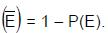• The odds in favor of occurrence of the event A are defined by m: n – m i.e., P (A): P (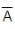) and the odds against the occurrence of A are defined by n – m : m, i.e., P (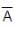): P (A)
• To find the probability of two or more independent events occurring in sequence, find the probability of each event occurring separately, and then multiply the answers.

Example:  If a number is selected at random from the 2 digit 6 multiples, what is the probability that it is divisible by 9?
Solution. Total 6 multiples = 12, - - - -, 96 ⇒ 15.
Required numbers are both multiples of 6 & 9
i. e, the multiples of 18. Those are 18, 36,- - - -, 90, total 5.
∴ Ans =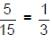Practice Question

Try yourself:Out of 13 applicants for a job, there are 5 women and 8 men. Two persons are to be selected for the job. The probability that at least one of the selected persons will be a woman is:

Example: If 2 dies are thrown, what is the probability of getting both the numbers prime?
Solution: P (Prime on first die) × P(Prime on second die)
=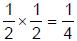Example: If 3 dies are thrown, what is the probability of getting a sum 4.
Solution: The possibility is 1, 1, 2 or 1, 2, 1 or 2, 1, 1.
∴ Ans =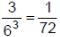Example: If 2 cards are drawn from a pack, what is the probability that both the cards are of different colours?
Solution: The possibility is 1 black and 1 Red.
i.e,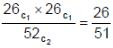Example: There are 5 red, 4 green, 3 yellow & 8 white balls in a bag, If three balls are chosen at random without replacement, what is the probability that they are of same color.
Solution: All the three can be red or green or yellow or white.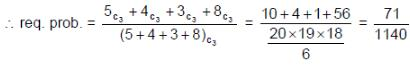Example: In a game, there are three rounds; the probabilities of winning first, second & third rounds are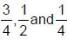respectively. A prize will be given, if any one wins all the rounds. What is the probability of winning the prize?
Solution: All the rounds have to be won to get the prize. So, the required answer is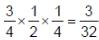.

Offer running on EduRev: Apply code STAYHOME200 to get INR 200 off on our premium plan EduRev Infinity!

## UPSC CSAT Preparation

44 videos|64 docs|90 tests

,

,

,

,

,

,

,

,

,

,

,

,

,

,

,

,

,

,

,

,

,

;